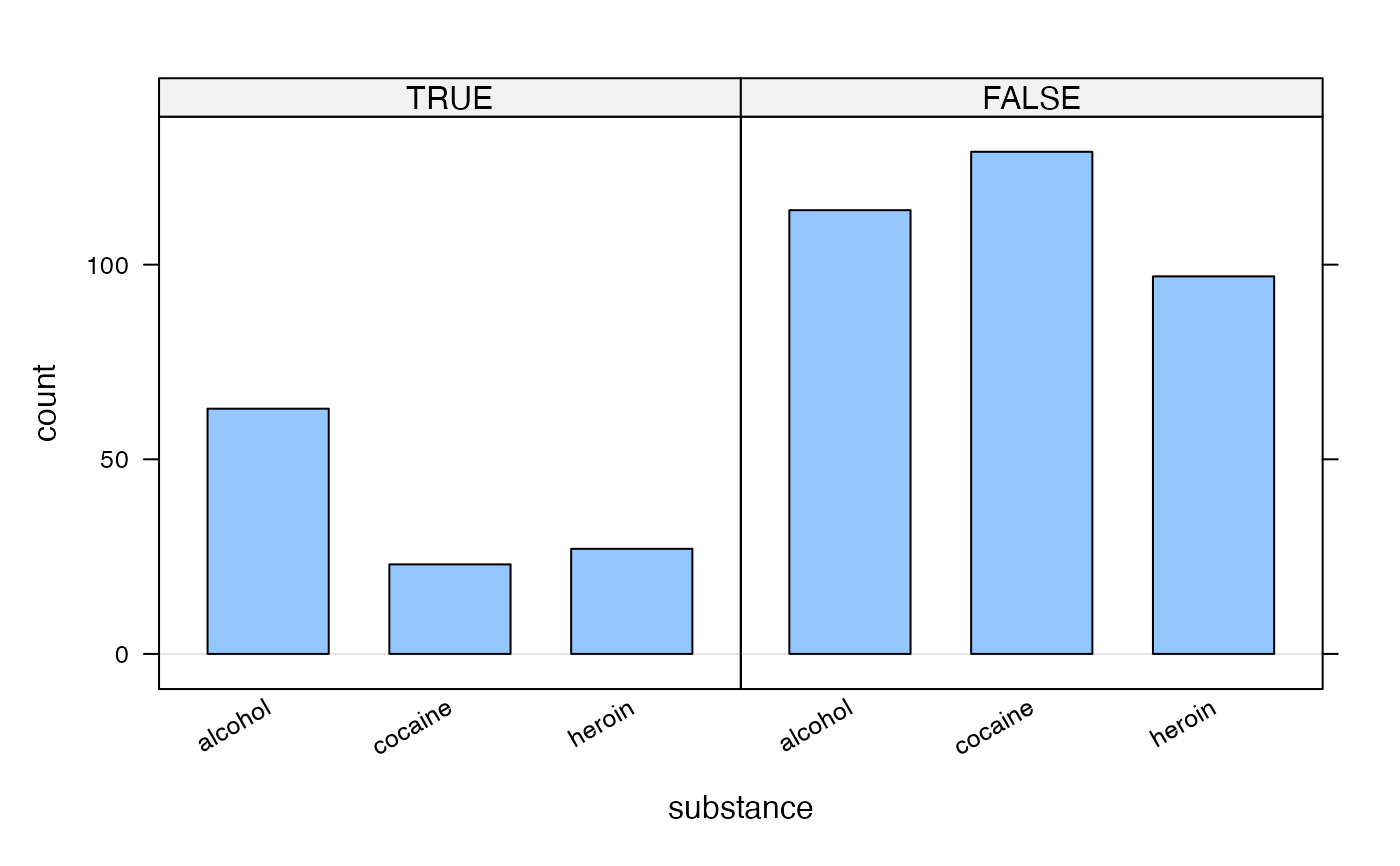lattice::barchart() from the lattice package makes bar graphs from pre-tabulated data. Raw data can be tabulated using xtabs(), but the syntax is unusual compared to the other lattice plotting functions. bargraph provides an interface that is consistent with the other lattice functions.

bargraph(
x,
data = parent.frame(),
groups = NULL,
horizontal = FALSE,
origin = 0,
ylab = ifelse(horizontal, "", type),
xlab = ifelse(horizontal, type, ""),
type = c("count", "frequency", "proportion", "percent"),
auto.key = TRUE,
scales = list(),
...
)

## Arguments

x

a formula describing the plot

data

a data frame in which the formula x is evaluated

groups

a variable or expression used for grouping. See lattice::barchart().

horizontal

a logical indicating whether bars should be horizontal

origin

beginning point for bars. For the default behavior used by lattice::barchart() set origin to NULL, but 0 is often a better default. If 0 is not good, perhaps you should use a different kind of plot as the results may be misleading.

ylab

a character vector of length one used for the y-axis label

xlab

a character vector of length one used for the x-axis label

type

one of "frequency", "count", "percent", or "proportion" indicating what type of scale to use. Unique prefixes are sufficient.

auto.key

a logical expression indicating whether a legend should be automatically produced

scales

is a list determining how the x- and y-axes are drawn

...

additional arguments passed to lattice::barchart()

## Value

a trellis object describing the plot

## Details

bargraph(formula, data=data, ...) works by creating a new data frame from xtabs(formula, data=data) and then calling lattice::barchart() using modified version of the formula and this new data frame as inputs. This has implications on, for example, conditional plots where one desires to condition on some expression that will be evaluated in data. This typically does not work because the required variables do not exist in the output of xtabs. One solution is to first add a new variable to data first and then to condition using this new variable. See the examples.

lattice::barchart()

## Examples

if (require(mosaicData)) {
data(HELPrct)
bargraph( ~ substance, data = HELPrct)
bargraph( ~ substance, data = HELPrct, horizontal = TRUE)
bargraph( ~ substance | sex, groups = homeless, auto.key = TRUE, data = HELPrct)
bargraph( ~ substance, groups = homeless, auto.key=TRUE,
data = HELPrct %>% filter(sex == "male"))
HELPrct2 <- mutate(HELPrct, older = age > 40)
bargraph( ~ substance | older, data = HELPrct2)
}